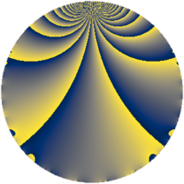# Properties

 Label 624.1.cbLevel $624$ Weight $1$ Character orbit 624.cb Rep. character $\chi_{624}(17,\cdot)$ Character field $\Q(\zeta_{6})$ Dimension $2$ Newform subspaces $1$ Sturm bound $112$ Trace bound $0$

# Related objects

## Defining parameters

 Level: $$N$$ $$=$$ $$624 = 2^{4} \cdot 3 \cdot 13$$ Weight: $$k$$ $$=$$ $$1$$ Character orbit: $$[\chi]$$ $$=$$ 624.cb (of order $$6$$ and degree $$2$$) Character conductor: $$\operatorname{cond}(\chi)$$ $$=$$ $$39$$ Character field: $$\Q(\zeta_{6})$$ Newform subspaces: $$1$$ Sturm bound: $$112$$ Trace bound: $$0$$

## Dimensions

The following table gives the dimensions of various subspaces of $$M_{1}(624, [\chi])$$.

Total New Old
Modular forms 32 6 26
Cusp forms 8 2 6
Eisenstein series 24 4 20

The following table gives the dimensions of subspaces with specified projective image type.

$$D_n$$ $$A_4$$ $$S_4$$ $$A_5$$
Dimension 2 0 0 0

## Trace form

 $$2 q - q^{3} + 3 q^{7} - q^{9} + O(q^{10})$$ $$2 q - q^{3} + 3 q^{7} - q^{9} + q^{13} - 2 q^{25} + 2 q^{27} - 2 q^{39} - q^{43} + 2 q^{49} - q^{61} - 3 q^{63} - 3 q^{67} + q^{75} - 2 q^{79} - q^{81} + 3 q^{91} - 3 q^{93} - 3 q^{97} + O(q^{100})$$

## Decomposition of $$S_{1}^{\mathrm{new}}(624, [\chi])$$ into newform subspaces

Label Dim. $$A$$ Field Image CM RM Traces $q$-expansion
$$a_2$$ $$a_3$$ $$a_5$$ $$a_7$$
624.1.cb.a $$2$$ $$0.311$$ $$\Q(\sqrt{-3})$$ $$D_{6}$$ $$\Q(\sqrt{-3})$$ None $$0$$ $$-1$$ $$0$$ $$3$$ $$q-\zeta_{6}q^{3}+(1+\zeta_{6})q^{7}+\zeta_{6}^{2}q^{9}-\zeta_{6}^{2}q^{13}+\cdots$$

## Decomposition of $$S_{1}^{\mathrm{old}}(624, [\chi])$$ into lower level spaces

$$S_{1}^{\mathrm{old}}(624, [\chi]) \cong$$ $$S_{1}^{\mathrm{new}}(156, [\chi])$$$$^{\oplus 3}$$